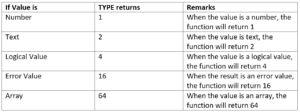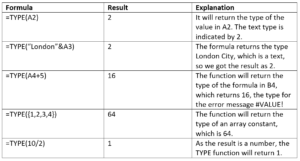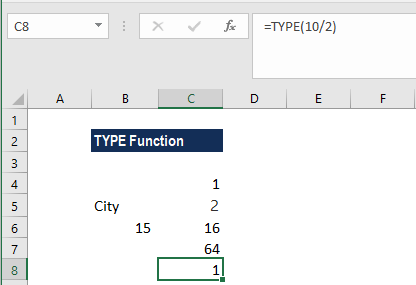# TYPE Function

Return the type of a given value

## What is the TYPE Function?

The TYPE function is categorized under Excel Information functions. It will return the type of a given value. The function will return a numeric code representing the type of value.

As a financial analyst, we use many Excel functions in our financial analysis. The TYPE function is useful when the behavior of another function depends on the type of value in a particular cell. If we are using functions that accept different types of data, TYPE can be used to find out what type of data is returned by a function or formula.

### Formula

=TYPE(value)

Value is a required argument. It is the value to check the type of. So, it can be any MS Excel value, such as a number, text, logical value, etc.### How to use the TYPE Function in Excel?

The TYPE Function was introduced in Excel 2007 and is available in all subsequent Excel versions. To understand the uses of the function, let us consider an example:

#### Example 1

Let’s see how the function will behave when we give the following formula:We get the results below:### A few things to remember about the TYPE Function

1. When we test value from a cell with a formula, the TYPE function will test the value returned by that formula.
2. If we refer to a cell that is blank, the function will return the number 1 as result.
3. If we refer to a cell that contains a date, it will return 1 as a result.

Thanks for reading CFI’s guide to important Excel functions! By taking the time to learn and master these functions, you’ll significantly speed up your valuation modeling. To learn more, check out these additional CFI resources:

• List of all Excel Functions